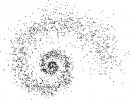#MATHZANI

## Squirrel numbersVous êtes ici : Accueil du site > My Maths > Squirrel numbers

### How it all started.

Today Nilo de Roock @ndroock1 tweeted the nice property 12²=144 and 21²=441 :

I added that 13 shared the same property and asked if there were any other numebr of this kind. I was on holiday but some followers such as @abnry, @jonpenguin or @JmFerrard programmed it and gave the list of the first numbers having this property. I propose to name these numbers squirrel numbers as a contraction of square & chiral.

### What’s a squirrel number ?

Definition (Chiral function). The chiral image of an integer written in some base is the number obtained when read from right to left in the same base. We’ll write X(n) the chiral image of integer n in base 10.Examples : X(123)=321, X(66)=66, X(69)=96, X(12321)=12321

Definition (Squirrel number). An integer n is said to be a squirrel number if n is not a palindrome (i.e. X(n)≠n) and it satisfies :

X(n²)=X(n

Examples : 12 and 13 are both squirrel numbers :12²=144 and 21²=441 so X(12²)=441=X(12)². Since 12≠21, 12 is a squirrel number.13²=169 and 31²=961 so X(13²)=961=X(13)². Since 13≠31, 13 is a squirrel number.We don’t want 10 to be considered as a squirrel even though X(10²)=X(100)=001=01². So I’m banning multiples of 10 from the list to avoid handling numbers starting with a 0.

Here is the list of squirrel numbers less than 10,000 :

12, 13, 21, 31, 112, 113, 122, 201, 211, 221, 301, 311, 1101, 1102, 1103, 1112, 1113, 1121, 1122, 1201, 1202, 1211, 1212, 1301, 2001, 2011, 2012, 2021, 2022, 2101, 2102, 2111, 2121, 2201, 2202, 2211, 3001, 3011, 3101, 3111
Or if you prefer to have them oredered by pairs :
(12, 21), (13, 31), (112, 211), (113, 311), (122, 221), (1102, 2011), (1103, 3011), (1112, 2111), (1113, 3111), (1121, 1211), (1122, 2211), (1202, 2021), (1212, 2121), (2012, 2102), (2022, 2202)

Apparently the squirrel numbers are not yet on OEIS so I’ll fill in a request.

These first squirrels were found using this program in Python. Below you’ll find the list of squirrels <100,000.

### Python code

Type squirrel(1000) to get every squirrel number less than 1000. Or sqp(1000) if you want them ordered by pairs.

``` #lnum(123)=[3,2,1] def lnum(n):  L=[n%10]  while n>10:    n=n//10    L.append(n%10)  return L #chir(n) returns the chiral image of a number eg chir(123)=321 def chir(n):  L=lnum(n)  j=len(L)  c=0  for i in range(j):    c=c+L[i]*10**(j-i-1)  return c #squirrel(N) returns the list of squirrel numbers <N. def squirrel(N):  LL=[]  for n in range(10,N):    if chir(n**2)==chir(n)**2 and n!=chir(n):      LL.append(n)  return LL >>> squirrel(100000) [10, 12, 13, 20, 21, 30, 31, 100, 110, 112, 113, 120, 122, 130, 200, 201, 210, 211, 220, 221, 300, 301, 310, 311, 1000, 1100, 1101, 1102, 1103, 1110, 1112, 1113, 1120, 1121, 1122, 1130, 1200, 1201, 1202, 1210, 1211, 1212, 1220, 1300, 1301, 2000, 2001, 2010, 2011, 2012, 2020, 2021, 2022, 2100, 2101, 2102, 2110, 2111, 2120, 2121, 2200, 2201, 2202, 2210, 2211, 3000, 3001, 3010, 3011, 3100, 3101, 3110, 3111, 10000, 11000, 11001, 11002, 11003, 11010, 11012, 11013, 11020, 11021, 11022, 11030, 11031, 11100, 11101, 11102, 11103, 11110, 11112, 11113, 11120, 11121, 11122, 11130, 11200, 11201, 11202, 11210, 11220, 11300, 12000, 12001, 12002, 12010, 12011, 12012, 12020, 12100, 12101, 12102, 12110, 12111, 12120, 12200, 12201, 12202, 13000, 13001, 13010, 13011, 20000, 20001, 20010, 20011, 20012, 20020, 20021, 20022, 20100, 20101, 20110, 20111, 20112, 20120, 20121, 20122, 20200, 20201, 20210, 20211, 20220, 20221, 21000, 21001, 21002, 21010, 21011, 21020, 21021, 21100, 21101, 21102, 21110, 21111, 21200, 21201, 21210, 22000, 22001, 22002, 22010, 22011, 22020, 22100, 22101, 22102, 22110, 22111, 30000, 30001, 30010, 30011, 30100, 30101, 30110, 30111, 31000, 31001, 31010, 31011, 31100, 31101, 31110, 31111] ```

@JmFerrard added this, which seems true from the examples above :

Now David Radcliffe @daveinstpaul mentionned that squirrels are just non-palindromic "skinny numbers" in A061909 "Skinny numbers : numbers n such that there are no carries when n is squared by "long multiplication"."